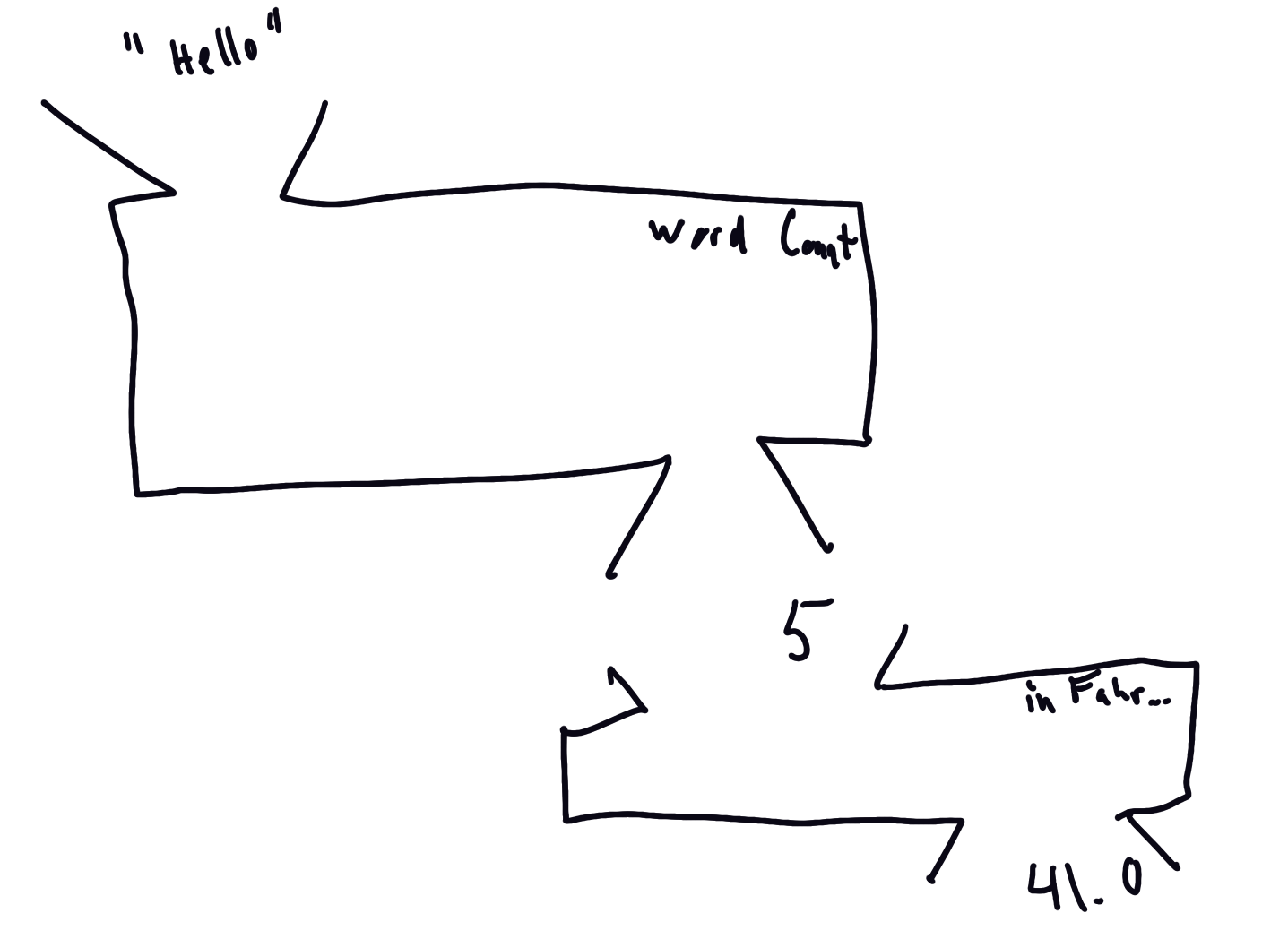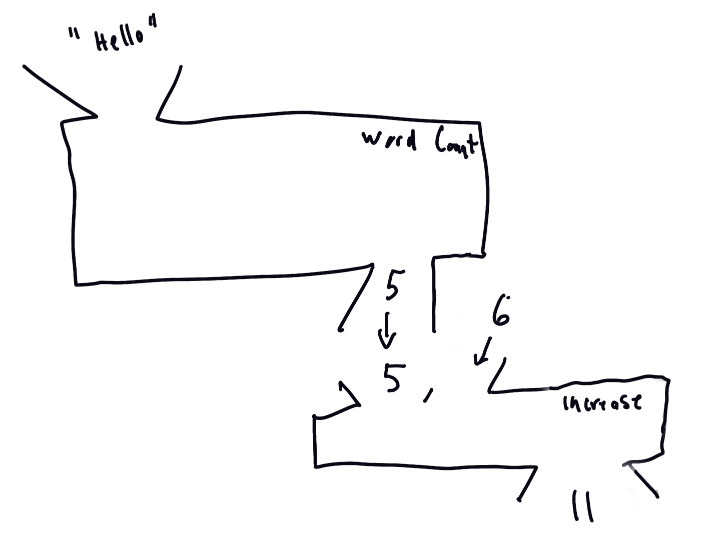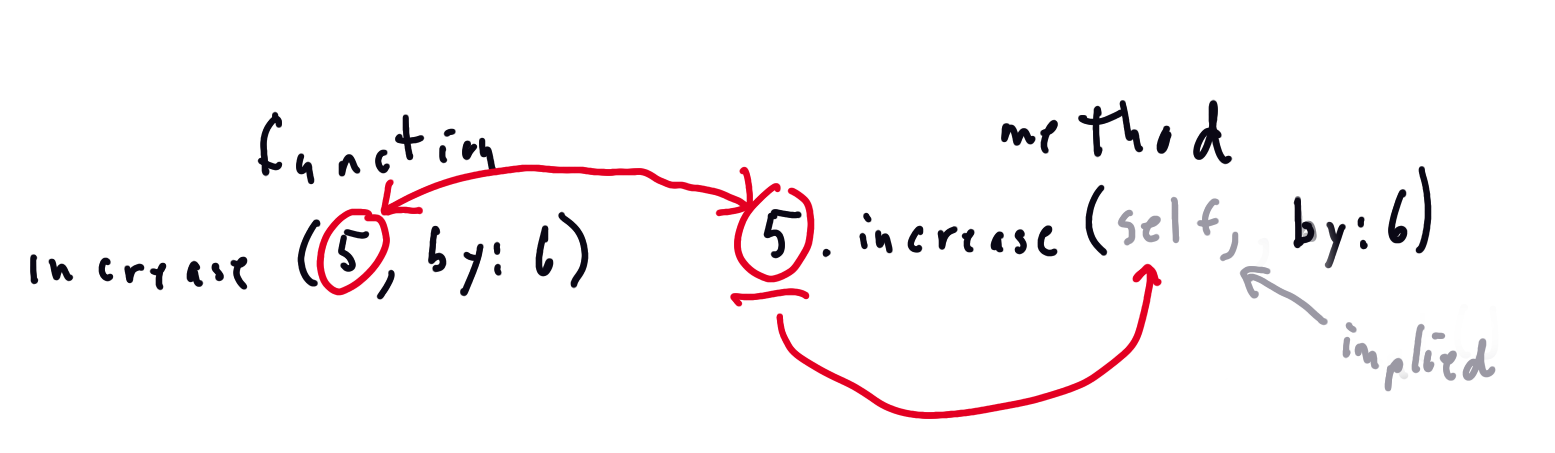## Methods and Functions

This month I’m inviting you to play along.

I’ve been working on a book about functional programming in Swift. To me that means “and methods too” but I’ve found that’s not obvious.

So in this brief post I want to look at methods vs functions both in who they are and in how we talk to them.

I know the classic image for a function but I’m not sure how to represent a method - particularly when it’s time to chain the methods. Your challenge is included at the end of this post.

Suppose I have a simple function that takes a `String` and returns an `Int`.

``````func wordCount(of string: String) -> Int {
string.count
}
``````

Then we call `wordCount()` like this:

``````wordCount(of: "Hello)
``````

Generally, we use a “function machine” to represent this. I used to teach High School Mathematics, and this is what we drew on the board to represent functions.

“Drew on the board?” you ask.

Yes. Search the internet for “blackboard” and “chalk” or ask an older person.

Anyway, the picture looked like this.Let’s represent composition next. `wordCount()` was a function `(String) -> Int` so the next function must take an `Int`.

As a silly example, suppose, because I live in the US, I want to convert my `wordCount()` result from Celsius to Fahrenheit. The conversion from C -> F is given by

``````func inFahrenheit(_ int: Int) -> Double {
Double(int) * 9 / 5 + 32
}
``````

So to calculate my word count in Fahrenheit, we have to compose these functions

``````inFahrenheit(wordCount("Hello")
``````

This works nicely in an image.So that works nicely for functions that take a single input. But what about functions that take two inputs.

For example, here’s a function that increases an `Int` by another `Int` amount. We could call it `add()`, I’ll leave it like this

``````func increase(_ int: Int,
by increment: Int) -> Int {
int + increment
}
``````

I’m less interested in the implementation than I am with the picture. But first let’s call the function.

``````increase(5, by: 6)
``````

And here’s how we compose it with `wordCount()`

``````increase(wordCount("Hello"), by: 6)
``````

The code is now harder to parse but the image still isn’t so bad.The first parameter falls through from `wordCount()` and we pass in the additional parameter

The code is cleaner and the methods are more discoverable if we use methods instead of functions.

Add `wordCount()` as a method in an extension to `String`.

``````extension String {
func wordCount() -> Int {
count
}
}
``````

We call it like this.

``````"Hello".wordCount()
``````

The argument of the function moves out front as the receiver of the message.

I know that “receiver” is very Objective-C of me but it feels more natural for me to think of it that way then the more formal “instance on which the method is called”.Similarly, we can define our other two functions as methods in an extension of `Int`.

``````extension Int {
func inFahrenheit() -> Double {
Double(self) * 9 / 5 + 32
}

func increase(by increment: Int) -> Int {
self + increment
}
}
``````

We call them as methods like this.

``````5.inFahrenheit()

5.increase(by: 6)
``````

Notice in this implementation that one of function’s parameters has come inside our method as `self`.

In other words if we use a function not a method then we have to explicitly pass the information contained in `self` in the method to the function.It is easy to chain methods in code than to compose functions (we’re not using custom operators here).

Here’s what chaining looks like.

``````"Hello"
.wordCount()
.inFahrenheit()

"Hello"
.wordCount()
.increase(by: 6)
``````

How should we represent methods and method chaining pictorially? We could just use the function diagrams and include the implied `self` but that doesn’t feel satisfying.

If you have an idea, please tweet with hashtag #drawingYOCmethods in the next couple of weeks.

Thanks.